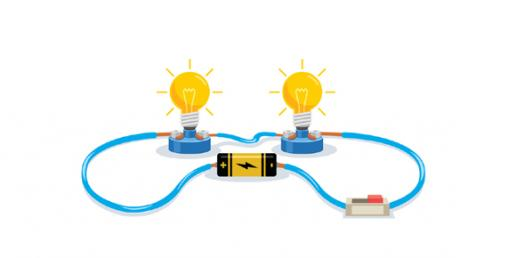# Electrical Circuits (B) - Quiz 1

70 Questions | Attempts: 472
ShareSettings.

• 1.
When two loops with or without contacts between them affect eachother through the magnetic field generated by one of them, they aresaid to be magnetically coupled.
• A.

True

• B.

False

• 2.
The resonant circuit consists only of a capacitor with a voltage or current source.
• A.

True

• B.

False

• 3.
This curve is for bandstop filter
• A.

True

• B.

False

• 4.
The resonant circuit consists only of inductor with a voltage or current source.
• A.

True

• B.

False

• 5.
This curve indicates that power willbe a maximum at fr and varying thefrequency in either direction resultsin a rise of the power.
• A.

True

• B.

False

• 6.
The value of ω that satisfies this condition is called the resonant frequency
• A.

True

• B.

False

• 7.
The bandwidth is the average between the half power points on the response curve of the filter.
• A.

True

• B.

False

• 8.
The total impedance of RLC parallel circuit is given in the shown relation
• A.

True

• B.

False

• 9.
The phase of The current across coil in series resonant circuit is +90 when the supply is 5Sinωt
• A.

True

• B.

False

• 10.
In RLC series resonance the Magnitude of inductive reactance must Eliminates the Magnitude of capacitive reactance to result a pure inductor
• A.

True

• B.

False

• 11.
Since the current is maximum at resonance,it follows that the power  must similarly be maximum at resonance.
• A.

True

• B.

False

• 12.
The power response of a series resonant circuit has a  bell-shaped curve called the selectivity curve.
• A.

True

• B.

False

• 13.
If the bandwidth of a circuit is kept very narrow , the circuit is said to have a Low selectivity
• A.

True

• B.

False

• 14.
The Bandwidth of the resonant circuit (BW) is The difference between the frequencies at which the circuit delivers half of the maximum power.
• A.

True

• B.

False

• 15.
The resonant frequency is the geometric mean of the half-power frequencies. The resonant frequency is the geometric sum of the half-power frequencies.
• A.

True

• B.

False

• 16.
The “sharpness” of the resonance in a resonant circuit is measured quantitatively by the quality factor Q.
• A.

True

• B.

False

• 17.
The quality factor of resonance circuit doesn't depent on resistance
• A.

True

• B.

False

• 18.
In series resonance, The higher the value of Q , the more selective the circuit is but more bandwidth.
• A.

True

• B.

False

• 19.
In series resonance, If the band of frequencies to be selected or rejected is narrow, the quality factor of the resonant circuit must be high.
• A.

True

• B.

False

• 20.
At parallel resonance, the admittance consists only conductance G = 1/2R.
• A.

True

• B.

False

• 21.
At parallel resonance,The value of current will be minimum since the total admittance is maximun
• A.

True

• B.

False

• 22.
At parallel resonance,The Power factor is unity.
• A.

True

• B.

False

• 23.
At parallel resonance, The currents through the inductor and the capacitor have the same magnitudes but are 90 out of phase.
• A.

True

• B.

False

• 24.
The magnitude of current in the reactive elements at parallel resonance is Q times Lower than the applied source current.
• A.

True

• B.

False

• 25.
In practical parallel resonance , if the internal resistance of the coil is negligable , so the resonance frequency of the circuit will be equal to the resonant frequency of ideal parallel resonance
• A.

True

• B.

False

## Related TopicsBack to top
×

Wait!
Here's an interesting quiz for you.